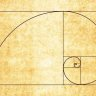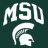# Donchian Channel with Fibonacci Zone Indicator for ThinkorSwim#### BenTen

Staff
VIP
Here is the converted version of Fibonacci Zone on TradingView to ThinkorSwim. According to the developer, it was a Donchian Channel but instead of using the median line he added four Fibonacci lines.How to read the Fibonacci Zone:

• Blue area is the uptrend zone
• Grey area is the ranging zone
• Orange area is the downtrend zone
• Outside of the mentioned zones is in-betweens

### thinkScript Code

Rich (BB code):
``````# WalkingBallista

input length = 21;

plot hl = highest(high, length);
plot ll = lowest(low, length);
def range = hl-ll;
plot hf = hl-range*0.236;
plot cfh = hl-range*0.382;
plot cfl = hl-range*0.618;
plot lf = hl-range*0.764;

DefineGlobalColor("Dark_Blue", createColor(0,0,100));
DefineGlobalColor("Dark_Orange", createColor(150,100,0));
DefineGlobalColor("Dark_Gray", Color.Dark_Gray);

cfh.setDefaultColor(Color.Dark_Gray);
cfh.setLineWeight(2);
cfl.setDefaultColor(Color.Dark_Gray);
cfl.setLineWeight(2);
hl.setDefaultColor(Color.Blue);
hl.setLineWeight(2);
hf.setDefaultColor(Color.Blue);
hf.setLineWeight(2);
ll.setDefaultColor(GlobalColor("Dark_Orange"));
ll.setLineWeight(2);
lf.setdefaultColor(GlobalColor("Dark_Orange"));
lf.setLineWeight(2);``````

https://tos.mx/apRWcv

Thanks to WalkingBallista for converting the script.

Last edited:
•#### Billions

##### Active member
2019 Donor
VIP
@BenTen Is this the same script

Code:
``````//@version=3

study(title = "Super-Trend-fib", overlay = true)

per=input(100, "calculate for last ## bars")
hl=highest(high,per) //High Line (Border)
ll=lowest(low,per)   //Low Line  (Border)
dist=hl-ll          //range of the channel
hf=hl-dist*0.236    //Highest Fibonacci line
cfh=hl-dist*0.382    //Center High Fibonacci line
cfl=hl-dist*0.618    //Center Low Fibonacci line
lf=hl-dist*0.764     //Lowest Fibonacci line
fill(plot(hl, title="high border"), plot(hf), color = orange) //up trend zone

fill(plot(ll, title="low border"), plot(lf), color=lime)   //down trend zone
//
//
use2bars=input(true,title="use 2 bars?")
lookback=input(100, type=integer, minval=1, maxval=100,title="look back bars?")
range = high-low+0.000000001                                                        // this way avoiding zero in the denominators
range2= (highest(high,2)-lowest(low,2))+0.000000001
value1=na
value1:= close > open and range != 0 ? (range/(2*range+open-close))*volume : value1
value1:= close < open and range != 0 ? ((range+close-open)/(2*range+close-open))*volume: value1
value1:= close == open ? 0.5*volume:value1                                       //value1 is up volume
value2 = volume-value1                                                           //value2 is down volume

//using only one bar
value3 = abs(value1+value2)
value4 = value1*range
value5 = (value1-value2)*range
value6 = value2*range
value7 = (value2-value1)*range

value8  = value1/range
value9  = (value1-value2)/range
value10 = value2/range
value11 = (value2-value1)/range
value12 = value3/range

// Using two bars
value13 = use2bars?( value3+value3):na
value14 = use2bars?(value1+value1)*range2:na
value15 = use2bars?(value1+value1-value2-value2)*range2:na
value16 = use2bars?(value2+value2)*range2:na
value17 = use2bars?(value2+value2-value1-value1)*range2:na

value18 = use2bars ? (value1+value1)/range2:na
value19 = use2bars ? (value1+value1-value2-value2)/range2:na
value20 = use2bars ? (value2+value2)/range2:na
value21 = use2bars ? (value2+value2-value1-value1)/range2:na
value22 = use2bars ? value13/range2:na

// one bar conditions
condition1 = value3 == lowest(value3,lookback)
condition2 = value4 == highest(value4,lookback) and close > open
condition3 = value5 == highest(value5,lookback) and close > open
condition4 = value6 == highest(value6,lookback) and close < open
condition5 = value7 == highest(value7,lookback) and close < open
condition6 = value8 == lowest(value8,lookback) and close < open
condition7 = value9 == lowest(value9,lookback) and close < open
condition8 = value10 == lowest(value10,lookback) and close > open
condition9 = value11 == lowest(value11,lookback) and close > open
condition10 = value12 == highest(value12,lookback)

// two bar conditions
condition11 = use2bars and value13 == lowest(value13,lookback)
condition12 = use2bars and value14 == highest(value14,lookback) and close > open and close > open
condition13 = use2bars and value15 == highest(value15,lookback) and close > open and close > open
condition14 = use2bars and value16 == highest(value16,lookback) and close < open and close < open
condition15 = use2bars and value17 == highest(value17,lookback) and close < open and close < open
condition16 = use2bars and value18 == lowest(value18,lookback) and close < open and close < open
condition17 = use2bars and value19 == lowest(value19,lookback) and close < open and close < open
condition18 = use2bars and value20 == lowest(value20,lookback) and close > open and close > open
condition19 = use2bars and value21 == lowest(value21,lookback) and close > open and close > open
condition20 = use2bars and value22 == highest(value22,lookback)

//Classifying the bars using one bar conditions, or using both one bar and two bar conditions simultaneosly if "use2bars" button selected.

lowvolume  = (condition1 or condition11)
climaxupvolume = (condition2 or condition3 or condition8 or condition9 or condition12 or condition13 or condition18 or condition19)
climaxdownvolume = (condition4 or condition5 or condition6 or condition7 or condition14 or condition15 or condition16 or condition17)
churnvolume =  (condition10 or condition20)
climaxchurnvolume =  (condition10 or condition20) and (condition2 or condition3 or condition4 or condition5 or condition6 or condition7 or condition8 or condition9 or condition12 or condition13 or condition14 or condition15 or condition16 or condition17 or condition18 or condition19)

//study("betterX",overlay=true)
trend=1
supplyline=high
supportline=low
trend:=barstate.isfirst?1:close>nz(supplyline)?1:close<nz(supportline)?-1:nz(trend)

supplyline:= trend==1?climaxdownvolume?highest(2): high>supplyline?high: supplyline:   climaxdownvolume?highest(2): supplyline

supportline:= trend==-1? climaxupvolume? lowest(2): low<supportline?low: supportline :   climaxupvolume?lowest(2):  supportline

trailing=trend==-1?supportline:supplyline
trailing1=trend==1?supportline:supplyline
plot(trailing,title="trailline",color=trend==1?blue:red,linewidth=3,transp=0)

up=crossover(trailing,close)
down=crossunder(trailing,close)
plotshape(up,title="up", color=blue, style=shape.arrowup, text="up",size=size.small, location=location.belowbar)
plotshape(down,title="down", color=red, style=shape.arrowup, text="down",size=size.small, location=location.abovebar)

// Super Trend Strategy

sLines = input(true, "Show Super-Trend Lines")
Factor1=input(1,type =float, minval=1,maxval = 100)
Factor2=input(2,type =float, minval=1,maxval = 100)
Factor3=input(3,type =float, minval=1,maxval = 100)
Pd1=10//input(10,minval=1,maxval = 100)
Pd2=10 //input(10,minval=1,maxval = 100)
Pd3=10 //input(10,minval=1,maxval = 100)

// ST1

Up1=hlc3-(Factor1*atr(Pd1))
Up2=hlc3-(Factor2*atr(Pd2))
Up3=hlc3-(Factor3*atr(Pd3))
Dn1=hlc3+(Factor1*atr(Pd1))
Dn2=hlc3+(Factor2*atr(Pd2))
Dn3=hlc3+(Factor3*atr(Pd3))

// ST1.2

TrendUp1=na
TrendUp2=na
TrendUp3=na
TrendUp1:=close>TrendUp1? max(Up1,TrendUp1) : Up1
TrendUp2:=close>TrendUp2? max(Up2,TrendUp2) : Up2
TrendUp3:=close>TrendUp3? max(Up3,TrendUp3) : Up3

TrendDown1=na
TrendDown2=na
TrendDown3=na
TrendDown1:=close<TrendDown1? min(Dn1,TrendDown1) : Dn1
TrendDown2:=close<TrendDown2? min(Dn2,TrendDown2) : Dn2
TrendDown3:=close<TrendDown3? min(Dn3,TrendDown3) : Dn3

Trend1 = na
Tsl1 = na
Trend2 = na
Tsl2 = na
Trend3 = na
Tsl3 = na
Trend1 := close > TrendDown1 ? 1: close < TrendUp1? -1: nz(Trend1,1)
Tsl1 := Trend1==1 ? TrendUp1: TrendDown1
Trend2 := close > TrendDown2 ? 1: close < TrendUp2? -1: nz(Trend2,1)
Tsl2 := Trend2==1 ? TrendUp2: TrendDown2
Trend3 := close > TrendDown3 ? 1: close < TrendUp3? -1: nz(Trend3,1)
Tsl3 := Trend3==1 ? TrendUp3: TrendDown3

// Plotting

linecolor = Tsl1 < Tsl2 and Tsl2 < Tsl3 ? red : Tsl1 > Tsl2 and Tsl2 > Tsl3 ? blue : orange

// Conditions

longCond = na
shortCond = na
longCond := crossover(close, Tsl3)
shortCond :=crossunder(close, Tsl3)

bgcolor(color = longCond ? green : shortCond ? red : na, transp = 60)
plotshape(longCond, title = "Buy Signal", text = "B", style=shape.triangleup, location=location.belowbar, color = black, editable = false, transp = 0)
plotshape(shortCond, title = "Sell Signal", text = "S", style=shape.triangledown, location=location.abovebar, color = black, editable = false, transp = 0)

Last edited by a moderator:
•mailbagman2000#### BenTen

Staff
VIP
@Trading51 The link to the original script was included in the source code. I don't think it's the same.

S

#### skynetgen

##### Well-known member
Not the same script. But works of same concept -
combines the Donchian Fib lines with supertrend to generate signal

•BenTen#### zeek

##### Active member
2019 Donor
@BenTen Is this the same script

I get the following error when trying to install this script:

Invalid statement: //@ at 1:1

Any idea why?#### Billions

##### Active member
2019 Donor
VIP
@zeek Yes thats a tradingview scriptDonchian Strategy seemingly wildly successful Strategies 35